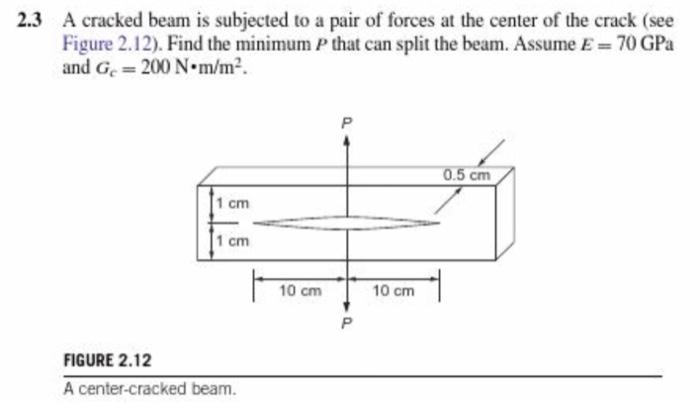# Question 2.3 A cracked beam is subjected to a pair of forces at the center of the crack (see Figure 2.12). Find the minimum P that can split the beam. Assume E= 70 GPa and Ge = 200 N·m/m². Р 0.5 cm 1 cm 1 cm 10 cm 10 cm FIGURE 2.12 A center-cracked beam.WWO8OB The Asker · Mechanical EngineeringTranscribed Image Text: 2.3 A cracked beam is subjected to a pair of forces at the center of the crack (see Figure 2.12). Find the minimum P that can split the beam. Assume E= 70 GPa and Ge = 200 N·m/m². Р 0.5 cm 1 cm 1 cm 10 cm 10 cm FIGURE 2.12 A center-cracked beam.
More
Transcribed Image Text: 2.3 A cracked beam is subjected to a pair of forces at the center of the crack (see Figure 2.12). Find the minimum P that can split the beam. Assume E= 70 GPa and Ge = 200 N·m/m². Р 0.5 cm 1 cm 1 cm 10 cm 10 cm FIGURE 2.12 A center-cracked beam.